# Spherical harmonics

(diff) ← Older revision | Latest revision (diff) | Newer revision → (diff)

The spherical harmonics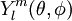$Y_l^m (\theta,\phi)$ are the angular portion of the solution to Laplace's equation in spherical coordinates. They are given by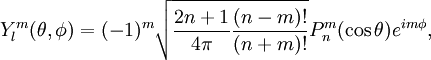$Y_l^m (\theta,\phi) = (-1)^m \sqrt{\frac{2n+1}{4\pi}\frac{(n-m)!}{(n+m)!}} P^m_n(\cos\theta) e^{i m \phi},$

where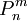$P^m_n$ is the associated Legendre function.

The first few spherical harmonics are given by: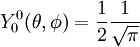$Y_0^0 (\theta,\phi) = \frac{1}{2} \frac{1}{\sqrt{\pi}}$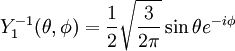$Y_1^{-1} (\theta,\phi) = \frac{1}{2} \sqrt{\frac{3}{2\pi}} \sin \theta e^{-i\phi}$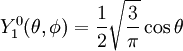$Y_1^0 (\theta,\phi) = \frac{1}{2} \sqrt{\frac{3}{\pi}} \cos \theta$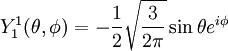$Y_1^1 (\theta,\phi) = -\frac{1}{2} \sqrt{\frac{3}{2\pi}} \sin \theta e^{i\phi}$